# Mean, Median, Mode, and Range Worksheet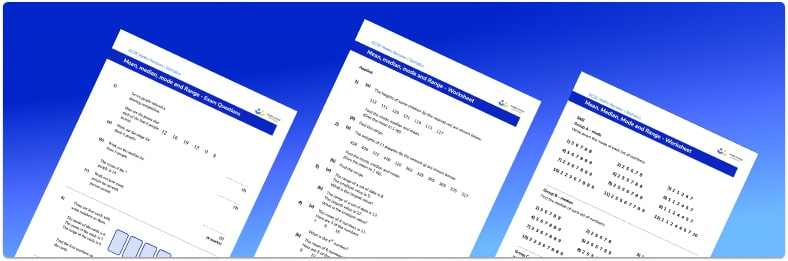Help your students prepare for their Maths GCSE with this free mean, median, mode, and range worksheet of 56 questions and answers

• Section 1 of the mean, median, mode, and range worksheet contains 48 skills-based mean median mode range questions, in 3 groups to support differentiation
• Section 2 contains 4 applied mean, median, mode, and range questions with a mix of word problems and deeper problem solving questions
• Section 3 contains 4 foundation and higher level GCSE exam style mean median mode range questions
• Answers and a mark scheme for all mean, median, mode, and range questions are provided
• Questions follow variation theory with plenty of opportunities for students to work independently at their own level
• All questions created by fully qualified expert secondary maths teachers

Suitable for GCSE maths revision for AQA, OCR and Edexcel exam boards

• To receive this resource and regular emails with more free resources, blog posts and other Third Space updates, enter your email address and click below.

• This field is for validation purposes and should be left unchanged.

You can unsubscribe at any time (each email we send will contain an easy way to unsubscribe). To find out more about how we use your data, see our privacy policy.

### Mean, median, mode, and range at a glance

Mean, median and mode are all measures of central tendency or average – each gives a central value to represent a set of data points.

The mode is the most frequently occurring value – this can be categorical or numerical. The mode is the only average that can be used for categorical data.

The median is the middle value when a set of numbers is put in order. For a small set of data, we write down the list of numbers in order, then systematically cross one number from each end in turn until we find the middle value. If there is an even number of values, this will result in two values identified as ‘the middle number’, so we state the mode as the midpoint of these two values. The mode can be a fraction or a decimal, even if the original data are integers.

To calculate the mean of a set of numbers, we add up all of the numbers in the set, then divide by how many numbers there are.

The average chosen depends on the set of data. For example, when comparing results on a test, when we talk about the average score, this tends to be the mean. However, if the data set contains a lot of extreme values or outliers, the median value may be more appropriate.

The range is often taught alongside mean, median and mode; it is important that students understand that the range is not an average, and instead is a measure of spread. To calculate range, we subtract the smallest value from the largest value in the data set.

Looking forward, students can then progress to additional Statistics worksheets, for example a line graph worksheet or pie chart worksheet.For more teaching and learning support on Statistics our GCSE maths lessons provide step by step support for all GCSE maths concepts.

## Related worksheets

X And Y Axis Worksheet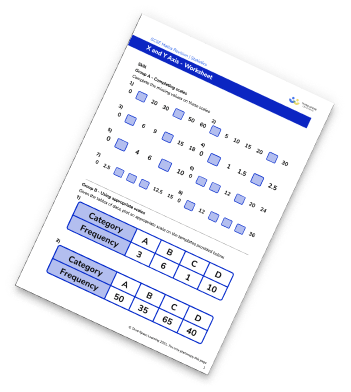Tally Charts Worksheet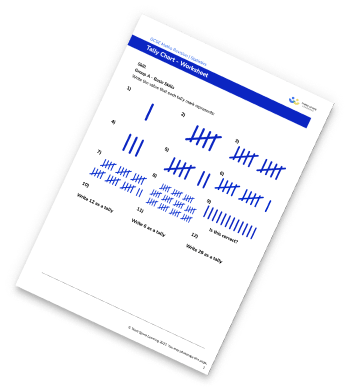Quartile And Interquartile Range Worksheet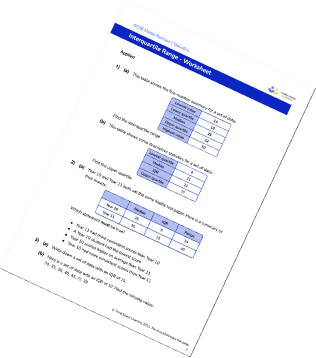Frequency graph worksheet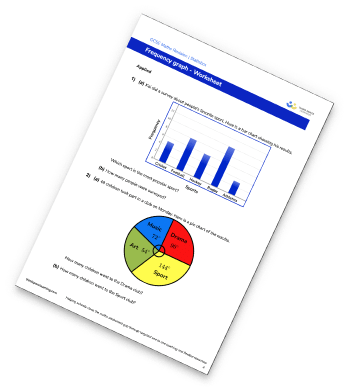## Do you have KS4 students who need more focused attention to succeed at GCSE?There will be students in your class who require individual attention to help them succeed in their maths GCSEs. In a class of 30, it’s not always easy to provide.

Help your students feel confident with exam-style questions and the strategies they’ll need to answer them correctly with our dedicated GCSE maths revision programme.

Lessons are selected to provide support where each student needs it most, and specially-trained GCSE maths tutors adapt the pitch and pace of each lesson. This ensures a personalised revision programme that raises grades and boosts confidence.

Find out more